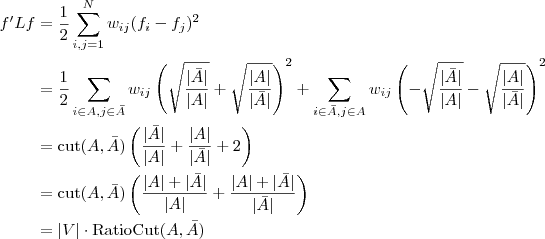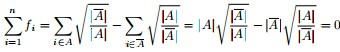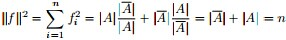# Kerighan-Lin算法

• 算法为类似模拟退火式的试探优化法，采用贪婪的策略对网络进行二分社区。其复杂度仅为O(N^2)，适用于小规模的网络，但准确度不高，并且必须事先知道两个社区的规模大小。
• 定义增益值$$P=\mbox{两个社区内部边数}-\mbox{两个社区之间边数}$$，并寻找使P最大的划分。
• 算法流程如下
• (1) 随机将整个网络中的节点划分为两个社区A和B，其节点数分别为m和n，m和n已知。
• (2) 对于A和B中的每对节点$$(i, j), i\in A, j\in B$$，计算将i和j交换后的$$\Delta P=\mbox{交换后的P}-\mbox{交换前的P}$$。
• (3) 选取使$$\Delta P$$最大的交换节点对并更新P值。另外，每个节点仅能交换一次。
• (4) 转(2)，直到A或者B二者中某个社区所有节点都已经被交换过一次。

# 基于Laplace图特征值的社区发现方法

• 一个无向图$$G=(V, E)$$，矩阵D是一个对角矩阵，对角线上的元素Dii是节点i的度，矩阵W是图G的邻接矩阵。拉普拉斯矩阵$$L=D-W$$，因此L为对称矩阵。其规定和具有的性质如下：

• 对于W，定义图中A、B两个子图间的权重为$$W(A, B)=\Sigma w_{ij}, i\in A, j\in B$$。
• 与某个节点邻接的所有边的权值和定义为该顶点的度，即$${di} = \Sigma w{ij}, i\in \lbrace 1, \ldots, N\rbrace$$。
• L是一个对称半正定矩阵。
• $$L \cdot \vec {1}=0 \cdot \vec {1}$$，因为$$L \cdot \vec {1}= (D-W) \cdot \vec {1} = 0 \cdot \vec {1}$$。
• L有n个非负实特征值$$0 = λ_1 \leq λ_2 \leq λ_3 \leq \ldots \leq λ_n$$。
• 对于任何一个实向量$$f\in R^n$$，有$$2 \cdot f’Lf=\Sigma w_{ij}\cdot (f_i - f_j)^2, i, j \in \lbrace 1, \ldots, N \rbrace$$。证明如下：$$f’Lf=f’Df-f’Wf=\Sigma d_i\cdot {f_i}^{2}-\Sigma {f_i}{fj}{w{ij}}(i, j\in {1, ldots, n})=\frac{1}{2}\cdot\left(\Sigma {d_i}{f_i}^{2}-2\Sigma {f_i}{fj}w{ij}+\Sigma {d_j}{fj}^{2}\right) (i, j\in {1, ldots, n})=\frac{1}{2}\cdot\left(\Sigma {w{ij}}({f_i}-{f_j})^{2}\right) (i, j\in {1, \ldots, n})$$
• 切割图的目的在于 使被切掉的各边之和最小 ，因为其代表着子图之间连接的相似度最低。

• 定义cut目标函数：$$cut(A1, A2, …, Ak) = \frac{1}{2} \cdot (\Sigma W(A_i, \overline{A_i}), i\in [1,k])$$。该目标函数可能会导致不好的分割，例如将某个图分成一个单一点和其余的n-1个点。
• 定义RatioCut目标函数：Ratiocut(A1, A2, ..., Ak) = (∑W(Ai, ~Ai)/|Ai|, i∈[1,k])/2。其中|Ai|代表社区i中的节点数目。 最小化RatioCut等价于最小化f’Lf ，这里的f = (f1, f2, ..., fn)∈R^n，并且当节点vi∈A时，fi = sqrt(|~A|/|A|)，否则fi = -sqrt(|A|/|~A|)。根据上面提过的拉普拉斯矩阵性质，有2f'Lf = ∑wij(fi - fj)^2, i,j from 1 to N。据此可以根据下面的推导得出，min f'Lf <=> min RatioCut• 因为向量f是单位向量1，所以有|f|^2 = ∑(fi^2) = n，且f'·1(单位向量）= ∑(fi) = 0。注意f是列向量，所以f'·f是值，而f·f'是一个NxN的矩阵。证明如下：• 假定L·f = λ·f，这里L是Laplace矩阵，λ是矩阵L的一个特征值，f是L对应λ的特征向量。同时左乘f’，得到f'·L·f = λn，因为n为定值，因此最小化f'·L·f等价于最小化λ。因此需要寻找最小的特征值λ和对应的特征向量。因为Laplace矩阵最小的特征值为0，因此取第二小的特征值。更进一步，如果求出拉普拉斯矩阵的前K个特征向量，进行k-Means聚类得到k个簇，就从二聚类拓展到了k聚类。
• 完整的算法描述如下
• 构造图W，将各数据点相连，边的权重表示数据间的相似度。
• 计算L = D - W（D为度矩阵，即W的每一列加到对角线上）
• 求L的前k个特征值{λ1, λ2, …, λk}，并且按从小到大顺序排序，求出对应的特征向量vi，每个特征向量是一个Nx1的列向量。
• 将这k个特征向量排成Nxk的矩阵，每一行都是k维空间中的一个向量，用k-Means聚类，聚类结果中的每一行的类别就是原来图中的节点所属类别。

# GN算法

• 1、计算每边的边介数，即网络上所有顶点对间的最短路径经过该边的次数。
• 2、移除最大介数边。
• 3、重新计算剩下边的介数。
• 4、重复步骤2，3，直到剩下的社区个数满足指定社区数目k。

# Newman快速算法

• 时间复杂度为O(m(m+n))，比GN算法优化较多。是凝聚算法。
• 首先将每个节点设为一个单独的社区，选出使模块度Q增值最大的社区合并，如果网络中所有顶点属于同一个社区则停止合并（自底向上的合并方式）。此时已经构造出了一棵凝聚树，这棵树的第k层对应着第k种社区划分方式，最底层对应着每个节点为一个社区。最终通过选取模块度最大的层数作为最佳划分。
• 模块度Q的计算如下：假设有n个节点，m条边，每一步合并对应社区数目为r，组成一个rxr的矩阵e，矩阵中eij表示社区i和社区j的结点之间连边的数目在整个网络边数中所占的百分比。
• 流程如下：
• 1、初始情况下，有n个社区，m条边，若社区i（节点i）与社区j有连边，则eij=1/(2m)，否则为0。
• 2、按照ΔQ最大或者最小的方向合并社区，并且更新合并后的模块度。这里增量ΔQ = eij + eji - 2aiaj = 2(eij - aiaj)，这里的ai = (∑eij)/2m
• 3、合并社区，并且修改矩阵e中的行列数。
• 4、重复步骤2、3，合并至树根。
• 5、计算模块度最大的社区划分。

# 派系过滤CPM方法

• 用于发现重叠社区，派系（clique）是任意两点都相连的顶点集合（完全子图）。k-派系表示网络中含有k个节点的完全子图。
• 社区内部节点之间相互联系密切，容易形成派系，因此社区内部的边有较大可能形成大的完全子图，而社区之间的边却几乎不可能形成较大的完全子图 => 从派系寻找社区。
• 首先寻找网络中的极大完全子图，利用这些完全子图来寻找k-派系的连通子图，不同的k值对应了不同的社区结构。
• 建立重叠矩阵：非对角元素代表两个连通派系中共享的节点数目，对角线元素代表派系的规模。将小于k-1的非对角线元素置为0，小于k的对角线元素置为1，得到k-派系连接矩阵。注意这里的k是输入参数，对结果有影响。k越大则生成的社区越大，社区的结构就越稀疏，通常k为4-6，视网络情况而定。
• CPM算法基于完全子图，因此适合完全子图比较多的网络，也就是边稠密网络，其处理稀疏图的效率较低。算法效率完全取决于寻找完全子图的效率，采用离线Tarjan算法会有所提高。

• 与GN相同，都基于去边，但不根据边介数，而引进边聚集系数，其算法复杂度为O(m^3/n^2)，适用于稀疏图。
• 边聚集系数： 一条边的两个端点和这两个端点的共同邻接点之间的另外两边所组成的三角环与可能包含该边的三角环数的比值，即：Cij = Zij/min(ki-1,kj-1)，这里ki，kj是端点i和j的度，公式中的分母表示该边可能被包含的三角环的最大数，Zij表示网络中包含该边的三角环的实际数目。
• 如果网络中的一个三角环中含有一条连接不同社区的边，则该三角环中剩余的两边中还有一条连接同样两社区的可能性较大（因为具有社区结构的网络图中，社区之间的边较为稀少，因此包含一条给定的社区间脸变得三角形不会很多，即连接不同社区边的边聚集系数很小）。 => 每一步删掉具有最小边聚集系数的边，并重新计算剩余边的边聚集系数（这里只需要重新计算和删除掉的边有关联的边的边聚集系数），循环至网络中不存在任何边。
• 算法仅适用于三角环数较多的，如社会网络等。

# 基于点聚集的局部算法

• 定义连接相关度：$$\lambda C(\varepsilon, j) = C{1}(j) - C{2}(j)$$，这里$$C{1}(j)$$指节点j的点聚集系数，$$C{2}(j)$$指去除社区$$\varepsilon$$内部的所有边以及与其相关联的所有边之后，节点j的点聚集系数。
• 定义点聚集系数：一个节点的不同邻接点互为邻接点的概率，公式为$$C(i) = \frac{2E(i)}{k{i}(k{i}-1)}$$，这里ki是节点i的度，E(i)是与节点i邻接的节点之间的实际连边。整个网络$$varepsilon$$的点聚集系数定义为$$C(ε) = \frac{\Sigma C(i)}{N}$$。
• 上面定义的连接相关度用来衡量某个社区ε对其邻接点的影响力大小。如果社区ε的一个邻接点x和x的邻接点间主要通过ε通信，则ε对x有重要影响，x趋向于成为ε的一员。
• 以下是社区归纳点的几个约定
• 如果节点x有一半以上的邻接点再ε中，则$$x\in \varepsilon$$。
• 如果$$C(\varepsilon)=1$$，这意味着ε和它的邻接点们构成连通分量，则该ε的所有邻接点∈ε。
• 如果该ε的一个邻接点j有C(j)=1，则j和j的邻接点都属于ε。
• 如果ε的邻接点j有C(j) > C(ε)。并且$$\Delte C(\varepsilon,j)$$是ε的所有邻接点中最大非负值，则$$j\in ε$$。
• 完整流程如下
• 以图中某个节点作为局部社区的初始状态，不断寻找j加入社区并update，直到没有符合条件的点，结束局部社区。
• 当所有局部社区形成后，分别计算每个社区的内度和外度，将内度小于外度的社区ε合并到与ε最紧密的社区中，直到所有社区都有内度>外度。
• 算法缺点是受到代表社区的初始节点影响比较大。

# 衡量网络分解：模块度

• 设网络分裂为g个社区，定义gxg的矩阵e，其eij表示原网络中连接社区i和社区j中节点的边数在所有边中所占比例。e的迹表示网络中同一社区中节点的边占所有边的比例。$$ai = \Sigma e{ij}$$表示连接社区i的边所占比例。有$$Q = Tre - {|e|}^2$$，$$\Sigma {ai}^{2} = \Sigma \left( \Sigma e{ij} \cdot e_{jk} \right) = {|e|}^2$$。